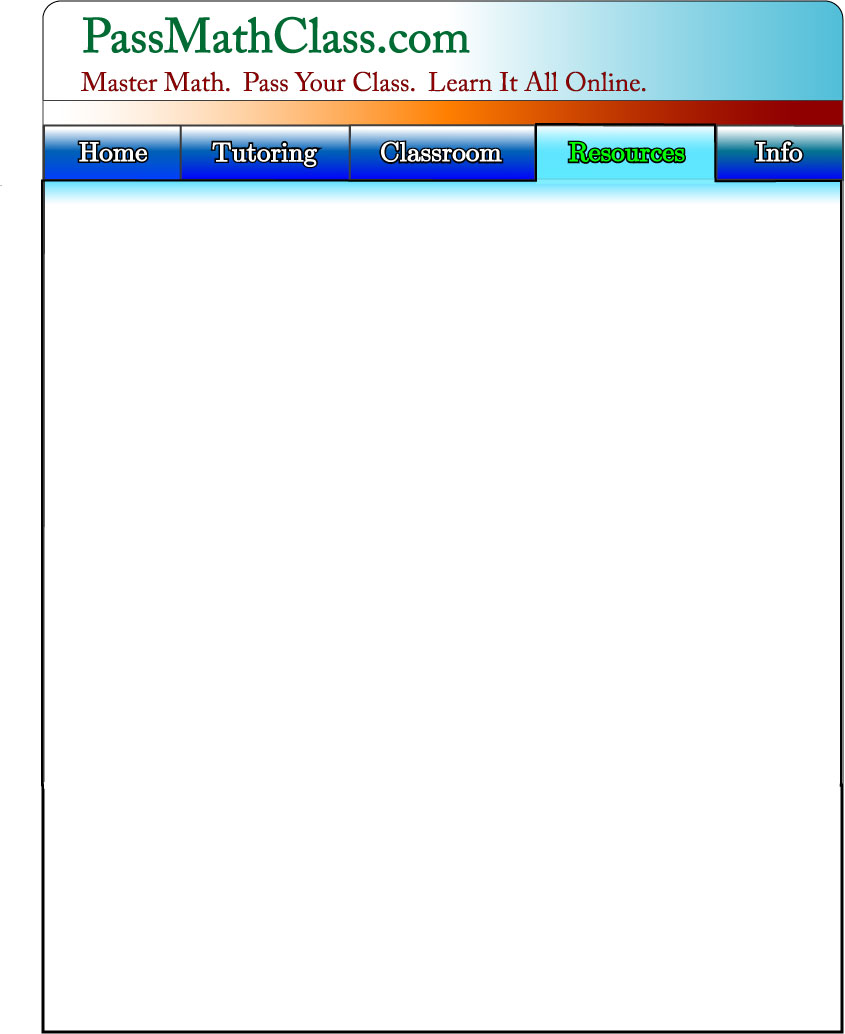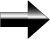Factoring

Factoring is one of the most important and most commonly used elements of algebra.  You will use factoring often.

Factoring is like the opposite of distributing.  In order to understand how to factor, we should look at how the function we are factoring was created.  That means becoming familiar with the FOIL method.

The FOIL Method

The FOIL method is the way that we multiply polynomials in algebra.  “FOIL” is an acronym for First, Outer, Inner, Last. Just remember the name "FOIL", and you'll remember how to apply it.

For example:  (x + 1)2 = (x + 1) * (x + 1) = (x * x) + (1 * x) + (x * 1) + (1 * 1) = x2 + 2x + 1.

Our knowledge of the FOIL method will help us in almost everything we will do in algebra and later math.

Main Algebra Page